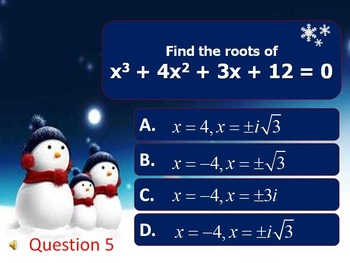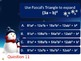# Alg 2 -- Polynomials & Polynomial FunctionsSubject
Resource Type
Common Core Standards
Product Rating
File Type

Compressed Zip File

40 MB
Share
Product Description
This powerpoint was created to review Chapter 6 of the Prentice Hall textbook for Algebra 2. It has twenty questions that cover these topics: polynomial functions; linear factors of polynomials; division of polynomials; solving polynomial equations; expanding binomials.

Because I like my students to work out each of the problems, rather than just guess, I have the question come up on the slide first. Then, after an appropriate time has passed, sleigh bells will ring to let the students know that "time is up." One click will bring up all four answers. One more click will cause the snowflake to float down to the correct answer.

All slide can be edited so this presentation can be modified to fit your classes. Math Type was used to create some of the questions and/or answers.

My students love these type of reviews which is why I have them for each of the chapters.

Common Core Standards:
A-APR
Use polynomial identities to solve problems.
5. (+) Know and apply the Binomial Theorem for the expansion of (x + y)n in powers of x and y for a positive integer n, where x and y are any numbers, with coefficients determined for example by Pascal’s Triangle.
Total Pages
N/A